# Acid, bases and saltsPage 7

#### WATCH ALL SLIDES

A pH of 4 is 10 times more acidic than a pH of 5.

A pH of 12 is 100 times more basic than a pH of 10.

Slide 42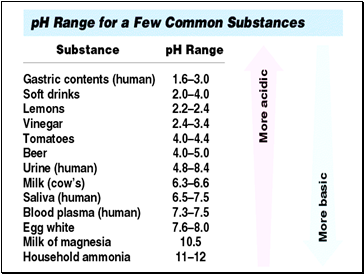Slide 43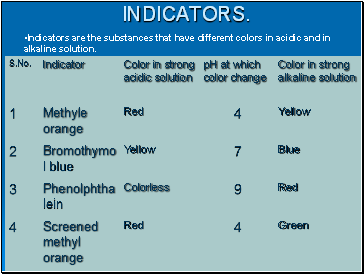## Indicators.

Indicators are the substances that have different colors in acidic and in alkaline solution.

Slide 44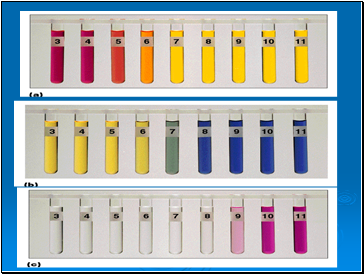Slide 45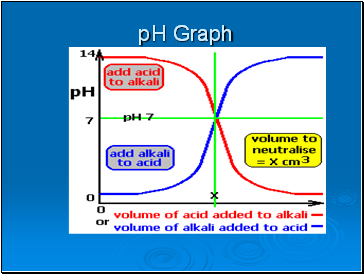## pH Graph

Slide 46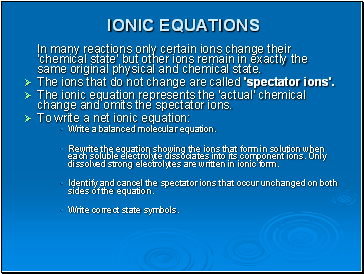## Ionic equations

In many reactions only certain ions change their 'chemical state' but other ions remain in exactly the same original physical and chemical state.

The ions that do not change are called 'spectator ions'.

The ionic equation represents the 'actual' chemical change and omits the spectator ions.

To write a net ionic equation:

Write a balanced molecular equation.

Rewrite the equation showing the ions that form in solution when each soluble electrolyte dissociates into its component ions. Only dissolved strong electrolytes are written in ionic form.

Identify and cancel the spectator ions that occur unchanged on both sides of the equation.

Write correct state symbols.

Slide 47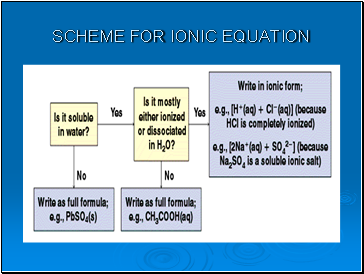## Scheme for ionic equation

Slide 48THE END

Go to page:
1  2  3  4  5  6  7